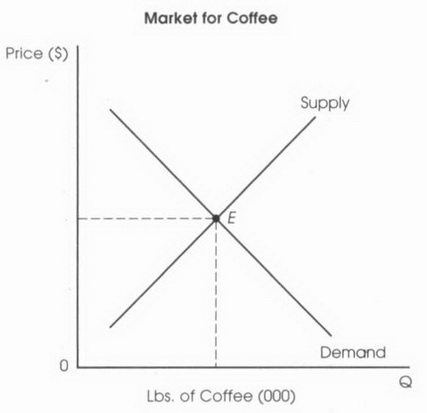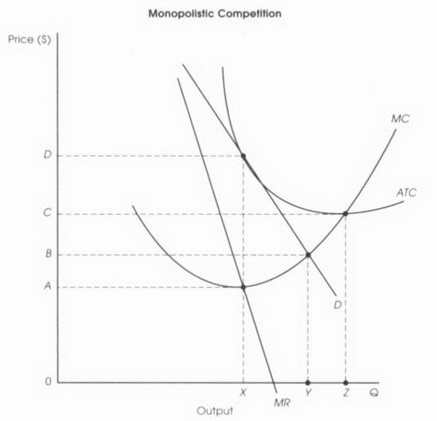# AP Microeconomics Practice Test 8

### Test Information12 questions14 minutes

1.Using the figure above, which of the following is correct for the market for coffee?

2.If the government provides a subsidy to the producers of coffee, which of the following will occur?

3.If the producers of coffee have to pay an increase in wages and fringe benefits to their workers, which of the following is correct?

4.If the price of coffee increases, which of the following is most likely to happen?

5. Which of the following is NOT a source of wage differentials?

6.For this firm operating under monopolistic competition, which of the following is the profit-maximizing output and price?

7.At the profit-maximizing output, which of the following is correct?

8. Which of the following best exemplifies economies of scale?

9. Which of the following is correct?

10. If a firm decreases its prices by 15 percent and its total revenue increases by 30 percent, which of the following is correct?

11. If one person has the only original signed copy of The Wealth of Nations by Adam Smith, which of the following would illustrate this situation?

12. When marginal cost equals price in a perfectly competitive product market at long run equilibrium, which of the following is NOT correct?